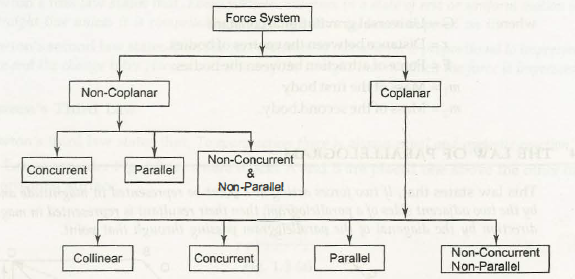Force can be explained as-

1. Pulling or pushing
2. It also explains about the tendency that can easily give a physical change in an object.
3. Force is the product of mass and acceleration of an object and thus F = ma.
4. Force can change the state of rest or state of motion of a body or tends to change.What are the different types of Forces?

The force system can be classified as –

• Non-coplanar – At the moment when the forces or system of forces act in the different parts of the body and which is not in a plane is known as non coplanar.
• Coplanar – At the time when the forces lie in the plane, then the systems are known as Coplanar system of forces.

Each type is divided in different parts as follows –

• Non-coplanar –
1. Concurrent
2. Parallel
3. Non-Concurrent and Non Parallel
• Coplanar –
1. Collinear
2. Concurrent
3. Parallel
4. Non-concurrent
5. Non-parallel

The various types say that when the forces act on a body is in the same line, and then it is called the collinear system of forces. When different forces intersect each other at a common point, then it is called concurrent forces. When the action of the forces is parallel, then it is called parallel forces.

Links of Next Mechanical Engineering Topics:-### Customer Reviews

My Homework Help
Rated 5.0 out of 5 based on 510 customer reviews at